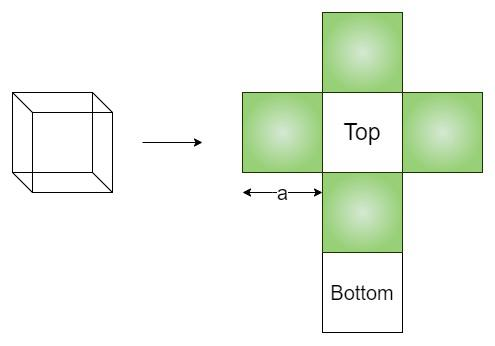GeeksforGeeks App
Open AppBrowser
Continue

# C++ Program to Find Curved Surface Area of a Cube

A cube is a 3-dimensional box-like figure represented in the 3-dimensional plane. Cube has 6 squared-shape equal faces. Each face meets another face at 90 degrees each. Three sides of the cube meet at the same vertex. The curved surface area of a cube is the sum of the areas of all its faces, except its top and bottom faces. Hence, the curved surface area of the cube is the sum of areas of all four side faces of a cube.Input: Side of the cube =2

Output: Curved Surface area = 16

Curved Surface Area of a cube is: 4 * a * a

where, a is the side of the cube

## C++

 `// C++ program to find area``// curved surface area of a cube``#include ``using` `namespace` `std;`` ` `// utility function``double` `CurvedareaCube(``double` `a)``{``    ``return` `(4 * a * a);``}``// driver function``int` `main()``{``    ``double` `a = 2;``    ``cout << ``"Curved surface area = "` `<< CurvedareaCube(a);``    ``return` `0;``}`

## Java

 `//Java program``// curved surface area of a cube``import` `java.io.*;` `class` `GFG {``  ``// utility function``  ``static` `double` `CurvedareaCube(``double` `a)``  ``{``    ``return` `4``*a*a;``  ``}``  ``// driver function``    ``public` `static` `void` `main (String[] args) {``      ``double` `a = ``2``;``      ``System.out.println(``"Curved surface area = "` `+CurvedareaCube(a));``        ` `    ``}``}` `//This code is contributed by suchethan`

Output

`Curved surface area = 16`

Time Complexity: O(1)

Auxiliary Space: O(1)

My Personal Notes arrow_drop_up